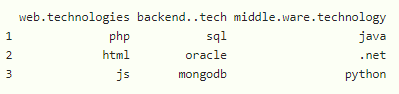Replace Spaces in Column Names in R DataFrame

• Last Updated : 23 Sep, 2021

In this article, we will replace spaces in column names of a dataframe in R Programming Language.

Let’s create a Dataframe with 4 columns with 3 rows:

R

 # create  a dataframe with 4 columns and 3 rowsdata = data.frame("web technologies" = c("php","html","js"),                  "backend  tech" = c("sql","oracle","mongodb"),                  "middle ware technology" = c("java",".net","python"))  # displaydata

Output:In the above example, we can see that there are blank spaces in column names, so we will replace that blank spaces

Method 1: Using gsub() Function

In this methods we will use gsub function, gsub() function in R Language is used to replace all the matches of a pattern from a string. If the pattern is not found the string will be returned as it is.

Syntax: gsub(” “, “replace”, colnames(dataframe))

Parameters:

• first parameter takes space
• second parameter takes replacing character that replaces blank space
• third parameter takes column names of the dataframe by using colnames() function

Example: R program to create a dataframe and replace dataframe columns with different synbols

R

 # create  a dataframe with 4 columns and 3 rowsdata=data.frame("web technologies"=c("php","html","js"),                 "backend  tech"=c("sql","oracle","mongodb"),                "middle ware technology"= c("java",".net","python"),                check.names=FALSE)        # replace blank with underscore             print( gsub(" ", "_", colnames(data)))    # replace blank with dot operator             print( gsub(" ", ".", colnames(data)))  # replace blank with * operator             print( gsub(" ", "*", colnames(data)))

Output:

 “web_technologies”       “backend__tech”          “middle_ware_technology”

 “web.technologies”       “backend..tech”          “middle.ware.technology”

 “web*technologies”       “backend**tech”          “middle*ware*technology”

Method 2: Using make.names() function

We can do this by using make.names() function.

Syntax: make.names(colnames(dataframe))

Where, dataframe is the input dataframe

Example: R program to replace dataframe column names using make.names

R

 # create  a dataframe with 4 columns and 3 rowsdata = data.frame("web technologies" = c("php","html","js"),                   "backend  tech" = c("sql","oracle","mongodb"),                  "middle ware technology" = c("java",".net","python"),                  check.names = FALSE)      # replace blank by using make.names             print( make.names(colnames(data)))

Output:

 “web.technologies”       “backend..tech”          “middle.ware.technology”

My Personal Notes arrow_drop_up